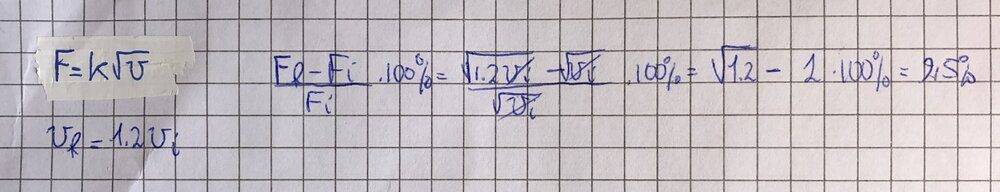# Percentage increase in resistance to motion

Gold Member
Homework Statement:
The resistance to the motion of a car is directly proportional to the square of the speed of the car.
The car increases its speed by 20%.
What is the percentage increase in the resistance to the motion of the car?
Relevant Equations:
Percentage increase;
So, I simply used the percentage increase formula for the resistance force, but what I come up with is a 9.5% increase, as opposed to the 44% it should be...
Here's my procedure:willem2
It's the square, not the square root.

•greg_rack
Gold Member
It's the square, not the square root.
What a fool! I'm Italian and understanding physics problems isn't always easy 😅... thank you!

Homework Helper
Gold Member
The resistive force to the movement of your car (and fuel consumption) always grows more rapidly than the speed does.
If the car increases its speed by 20%, naturally the percentage increase in the resistance to its motion should be 44%.

•greg_rack